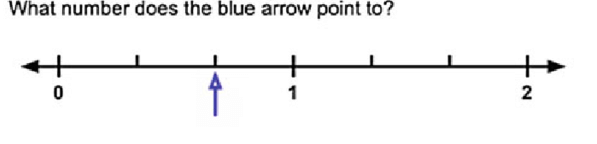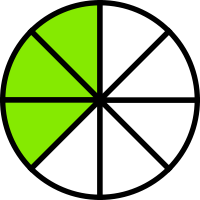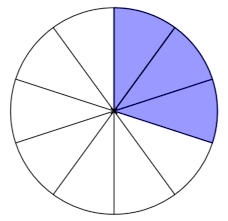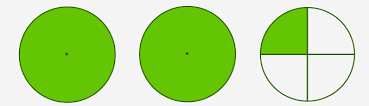Multiplication
Division
Fractions
Geometry
Subtraction
100

Name one factor in 6 x 5 = 30

6 or 5

100

64 divided by 8

8

1002/3

100

Trapezoid, square, rectangle, rhombus, parallelogram

100

3,432 - 2,961=

471

200

Name the product in 8 x 3 = 24

24

200

72 divided by 9

8
200

What fraction is NOT shaded?5/8

200

An angle is less than 90 degrees?

acute

200

631,453-286,349=

345,104

300

12 x 9=

108

300

99 divided by 11

9

300

What fraction of the circle is purple?3/10

300

A triangle with 3 equal sides.

equilateral triangle

300

1,000-734=

266

400

12 x 12=

144

400

121 divided by 11

11

400

What does this mixed number show?2 1/4

400

A line with 2 endpoints.

line segment

400

20,040-13,683=

6,317

500

15 x 4=

60

500

132 divided by 12

11

500

1/2+1/2=

1

500

What is a 12 sided shape called?

dodecagon
500

Bob went to the store and bought a Snickers and a soda. Each item costs $3.50. If he gives the cashier a$10 bill, how much change will he receive?

\$3

Click to zoom